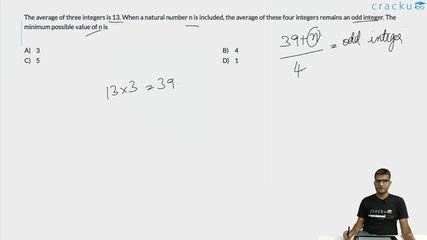Question 14

# The average of three integers is 13. When a natural number n is included, the average of these four integers remains an odd integer. The minimum possible value of n is

Solution

It is given that average of three numbers is 13.

Sum = 3*13 = 39

It is given, $$\ \frac{\ 39+n}{4}$$ is a odd number.

Minimum value $$\ \frac{\ 39+n}{4}$$ can take such that n is a natural number is 11

$$\ \frac{\ 39+n}{4}=11$$

n = 5

### View Video Solution##### Charul Chandwani

4 months, 2 weeks ago

How have we come down to the number 11, did not really understand it

##### Sreepurna Saha

3 months, 2 weeks ago

Find a number that is divisible by 4 and gives a odd vale.. so if you keep 40 as n it will give product as 10 but if you keep it as 44 it will give product 11 which is odd